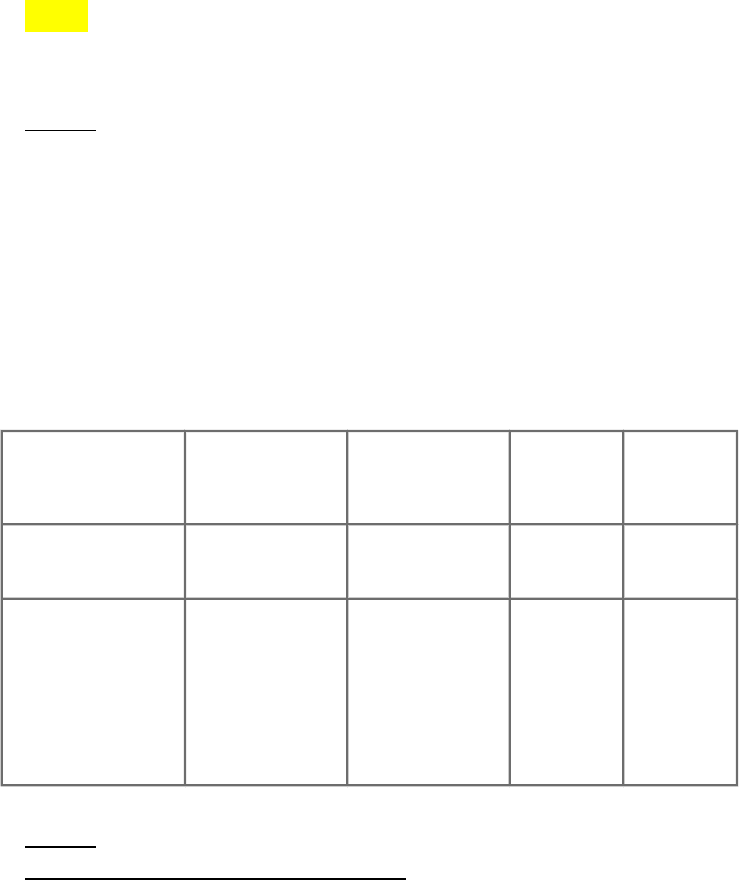Class Notes (1,000,000)
US (430,000)
UF (4,000)
STA (40)
Lecture 1

STA 3024 Lecture Notes - Lecture 1: Cumulative Distribution Function, Dependent And Independent Variables, Regression Analysis

Department
Statistics
Course Code
STA 3024
Professor
Ripol Maria
Lecture
1

This preview shows pages 1-3. to view the full 18 pages of the document.Statistics 2 Exam 2: Study Guide
1. Compute the intercept of the regression line if xbar= 66, ybar = 254, sy= 39, Sx
= 10 and r = 0.324.
-5.224
1.2636
170.6
0.083
Hint:
Slope: r * (sy/sx)
Y Intercept: y-bar – (slope * x-bar)
2. A study of nutrition in developing countries collected data from the Egyptian
village of Nahya. Here are the mean weights (in kilograms) for 170 infants in
Nahya who were weighed each month during the first year of life. A part of the
Minitab output is below. Find the 95% confidence interval for the slope. n=12
The regression equation is Weight = 4.88 + 0.267 Age.
Predictor Coef SE Coef T p-value
Constant 4.8803
(Alpha) 0.2895 16.86 0.000
Age
0.26713
*Sample
Statistic
0.03933
*Standard
Error
6.79 0.000
Hint:
To find the confidence interval:
Find standard deviation or standard error. The standard error is given in the
regression output. (SE coefficient: .0393)
Find critical value. The critical value is a factor used to compute the margin of
error. With simple linear regression, to compute a confidence interval for the slope,

Only pages 1-3 are available for preview. Some parts have been intentionally blurred.Statistics 2 Exam 2: Study Guide
the critical value is a t score with degrees of freedom equal to n - 2. To find the
critical value, we take these steps.
Compute alpha (α): α = 1 - (confidence level / 100) = 1 -
95/100 = 0.05
Find the critical probability (p*): p* = 1 - α/2 = 1 - 0.05/2
= 0.975
Find the degrees of freedom (df): df = n - 2 = 12 - 2 = 10.
The critical value is the t score having 10 degrees of
freedom and a cumulative probability equal to 0.975. From the t
Distribution Calculator, we find that the critical value is _____.
Compute margin of error (ME): ME = critical value * standard
error = ___ * .0393= ____
Specify the confidence interval. The range of the confidence interval is defined
by the sample statistic + margin of error. And the uncertainty is denoted by the
confidence level.
(4.24, 5.53)
(0.1795, 0.355)
(-1.65, 2.27)
(4.31, 5.45)
3. Compute the intercept of the regression line if x-bar = 50, y-bar = 268, r= 0.335,
sy = 39, sx = 10.
202.7
1.7956
1.3065
-429.72
4. To test if x is a good predictor of y in Simple Linear Regression we need to
determine whether:
-The slope is significantly different from zero.
5. In the Fall STA 2023 Beginning of the Semester Survey, students were asked
how many parties they attended every week and how many text messages they sent
per day. The researcher decided to make the number of parties attended per week
the explanatory variable and the number of text messages sent per day the response
variable. The least squares regression line for this relationship is y-hat = 64.96 +

Only pages 1-3 are available for preview. Some parts have been intentionally blurred.Statistics 2 Exam 2: Study Guide
25.41x. One student attended 3 parties that week and sent 200 text messages per
day. What is the residual?
Hint:
To find the residual.
1.) Label obs x and obs y.
2.) Find pred y by plugging obs x into the formula.
3.) Find the residual by taking obs y - pred y.
4946.96
-106.63
58.81
96.63
5146.96
-96.63
6. A political scientist was interested in studying America's voting habits. So, he
decided to make a least squares regression equation to predict the percentage of
people in a state that would vote for Obama in 2012 based on the percentage of
people in a state that voted for Obama in 2008. The least squares equation is y-hat
= -4.599 +1.04x. In the state of Wyoming, 32.54% voted for Obama in 2008;
whereas, 27.82% voted for him in 2012. What is the value of the residual?
3.3
-1.42
1.42
29.24
-3.3
7. The Leaning Tower of Pisa is an architectural wonder. The tower started to lean
during construction, which started in the year 1176 and continued for about 200
years. Engineers concerned about the tower's stability have done extensive studies
of its increasing tilt. Measurements of the lean of the tower represent the difference
between where a point on the tower would be if the tower were straight and where
it actually is. The data are coded as tenths of a millimeter in excess of 2.9 meters,
so a value of 642 on the graph corresponds t,o a lean of 2.9642 meters. The
measurements in this study were taken from 1975 to 1987, so n=13 years and only
the last two digits of the year were entered into the computer. Find the 99%
confidence interval for the slope.
The regression equation is Lean = -61.1 +9.32 year.